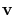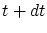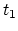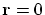Next: The concept of mass Up: Dynamics of the SRT Previous: Introduction   Contents

# Notions of relativistic dynamics

Now we shall proceed to a more complicated problem of dynamical concepts of SRT. It would seemed that only in the relativistic kinematics there are no direct experimental comparisons of physical quantities (only doubtful interpretations) for two systems moving relative to each other; but in the relativistic dynamics everything is in order (according to relativists' logic - the accelerators are operating, in fact!). Let us try to clear up the dynamical concepts, even because the relativistic dynamics, under modern interpretation of SRT apologists, rests upon a completely untrue relativistic kinematics.

We begin with general notes. A boundless spreading of the idea of relativity of all quantities in SRT is completely groundless. Really, let the two bodies be at distanceapart of each other while having relative velocity. Then the result of interaction of these bodies at instantwill not be determined by mentioned characteristics, but will depend on the prehistory of motion. Since the effect spreads at finite velocity, the first body at instantwill be influenced not by the real second body (at instant) with its coordinates and velocity, but by some its "image" from a preceding point of the trajectory, from which the effect had time to come before instant. Thus, any physical quantity (the force, for instance) can not depend on the relative velocity at the same instant only. The only exception is the frontal collision, at which. Therefore, it is necessary either to apply more complicated equations instead of the local differential equations (i.e. to take into account the prehistory), or to refuse from the idea of relativity of all quantities. Even the notion of the "relative velocity at the given time instant" itself becomes indefinite, because any real effect will be determined by characteristics at preceding instants. And, you see, SRT does not "know" the absolute velocity organically (it "knows"only the relative one). This fact has already resulted in the discomfiture. For example, Einstein has actually believed the stellar aberration to depend on the relative velocity of the Earth and a star (see , v.1). However, the experiment shows the stellar aberration to be dependent on the Earth velocity only, but the velocity of a star has no effect at all. In spite of vast scattering of velocities of stars, the aberration on the Earth is found to be the same for all stars. Where has the relative velocity gone in such a case? Actually, even this fact disproves the original concept of SRT. A similar disproof of SRT is obtained in the problem on a coil in the magnetic field: the motion of a coil induces the current in it immediately, whereas the motion of a magnet (according to the finiteness of the rate of interactions) - only after some time. There is no symmetry of the problem, and the dependence on the relative velocity only is obviously insufficient.

SubsectionsNext: The concept of mass Up: Dynamics of the SRT Previous: Introduction   Contents
Sergey N. Arteha